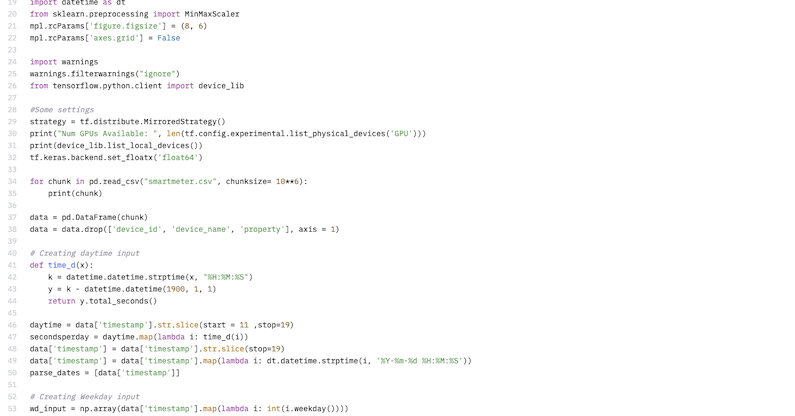# Democratizing neuronal networks to predict energy consumption

Vatsal ShaMay 15, 2023

We recently released LSTEnergy [last energy], a Long-Term-Short-Memory energy forecasting model which was trained using real-world smart meter data we captured in 2020, and trained using Blossom Sky. LSTEnergy is available in our GitHub repository  and in our HuggingFace space .

## Why we created a industry specific model

LLM and LSTM-based models are a big part of the current hype around AI, fueled by OpenAI and ChatGPT. Companies are starting to develop AI strategies, often without really knowing what they should do or how. Using large-scale models, which are powerful but also not specialized, often brings more confusion due to the fact that they are language-based models; they just provide conversations. Now, conversations aren't really helpful when a company wants to improve certain processes with AI.

With LSTEnergy, we want to help enterprises better understand their energy consumption, predict it much better, and therefore save energy and CO2. LSTEnergy is a time-series forecasting model that uses time-based historical data to predict future outcomes. Using Blossom Sky, this model is able to run on multiple, independent data stores or data lakes in a sliding time window approach to predict possible future consumption.

## Short overview how LSTEnergy works

LSTEnergy in a nutshell:

• initialize the LSTM model with 50 hidden units and 0.2 dropout rate
• train the model for 100 epochs with a batch size of 32 and validate it on the testing set
• using matplotlib to plot the training and validation loss curves
• generate predictions on the testing set and plot them against the actual values
• calculate the root mean squared error (RMSE) and the mean absolute percentage error (MAPE) of the predictions

LSTEnergy performs with high probability after approximately 20 epochs, depending on the dataset used. In a typical scenario, the model runs once a week per smart meter. To use LSTM for our time series forecasting, we need to train it on a dataset of historical data and try to find future values. Our LSTEnergy model learns a function that maps a sequence of past observations as input to an output observation, using the smartmeter dataset.

We have a dataset of energy consumption data captured over a longer period of time. We now train LSTEnergy to learn a function that maps a sequence of past consumption in x = days as input to an output of prognostic energy consumption. For example, we can use the last 10 days' consumption as input and predict the 11th day's consumption as output.

## Why we used a LSTM approach

LSTM (Long Short-Term Memory) is a special type of a recurrent neural network (RNN), that is capable of learning long-term dependencies. LSTM models have a special architecture, they use memory cells and gates to regulate the flow of information. This allows them to remember important information from the past while forgetting irrelevant information. They are extremely useful for time series based forecasting, where the goal is to predict future values based on past events.

In the context of time series forecasting, an LSTM model takes as input a sequence of past observations and outputs a prediction for the next value in the sequence. The model is trained on historical data to learn the underlying patterns and relationships between the input features and the target variable. When the model is successfully trained, it can be used to create predictions based on new data sets without being trained again. To improve the accuracy of LSTEnergy, a user can tune the number of layers or how many neurons per layer should be used.

Now, LSTM belongs to the family of neuronal networks. But RRNs tend to forget information that is too far back in the past. This is because the hidden state vector gets diluted by repeated multiplications and additions as it passes through the network. This problem is known as the "vanishing gradient", and it limits the ability of RNNs to learn long-term dependencies.

LSTM solves this problem by introducing a new component: a cell state vector c_t. The cell state acts as a memory that can store and retrieve information over long time spans. It is regulated by three gates: an input gate i_t, an output gate o_t, and a forget gate f_t. These gates are neural networks that learn to control what information to keep or discard from the cell state and the hidden state.

The input gate decides what new information to add to the cell state based on the current input x_t and the previous hidden state h_t. The forget gate decides what old information to erase from the cell state based on the same inputs. The output gate decides what information to output from the cell state based on the updated cell state c_t and the previous hidden state h_t.

The following equations describe how these gates work mathematically:

i_t = sigmoid(W_i * [h_(t-1), x_t] + b_i)

f_t = sigmoid(W_f * [h_(t-1), x_t] + b_f)

o_t = sigmoid(W_o * [h_(t-1), x_t] + b_o)

g_t = tanh(W_g * [h_(t-1), x_t] + b_g)

c_t = f_t * c_(t-1) + i_t * g_t

h_t = o_t * tanh(c_t)

y_t = softmax(W_y * h_t + b_y)

where W_i, W_f, W_o, W_g, and W_y are weight matrices, b_i, b_f, b_o, b_g, and b_y are bias vectors, sigmoid is a logistic function that squashes values between 0 and 1, tanh is a hyperbolic tangent function that squashes values between -1 and 1, and softmax is a function that normalizes values into a probability distribution. By using these gates, LSTM can learn to selectively store and retrieve relevant information from the cell state over long time spans. This allows it to capture long-term dependencies and avoid vanishing gradients.